LOADING

### Type to search# Dplyr Package For Data Manipulation in R

To Know more about the Different Corporate Training & Consulting Visit our website www.Instrovate.com Or Email : info@instrovate.com or WhatsApp / Call at +91 74289 52788

# Dplyr Package For Data Manipulation in R

##### ShareHadley Wickham released the dplyr package in January 2014. It is a powerful R-package for data manipulation , clean and summarize unstructured data. It makes data exploration and data manipulation easy and fast in R.

It contains some important functions(or verbs) –

Functions                Description

select()                   Select columns

filter()                     Filter rows

arrange()                Re-order or arrange rows

mutate()                 Create new column

summarise()           Summarise values

group_by()              Allows for group operations

For this tutorial , I am using the airquality dataset from the datasets package. The airquality dataset contains information about air quality measurements in New York from May 1973 to September 1973.

To install the “dplyr” package, type the following command :

install.packages(“dplyr”)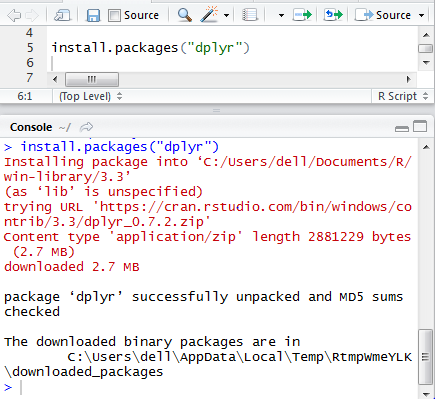Load “dplyr” package :

library(dplyr)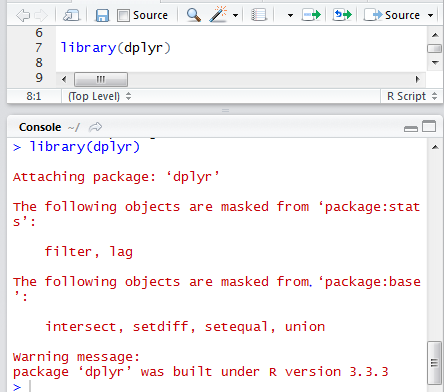We can check built-in R data by using following command :

data()

It can show all the data in “datasets” package.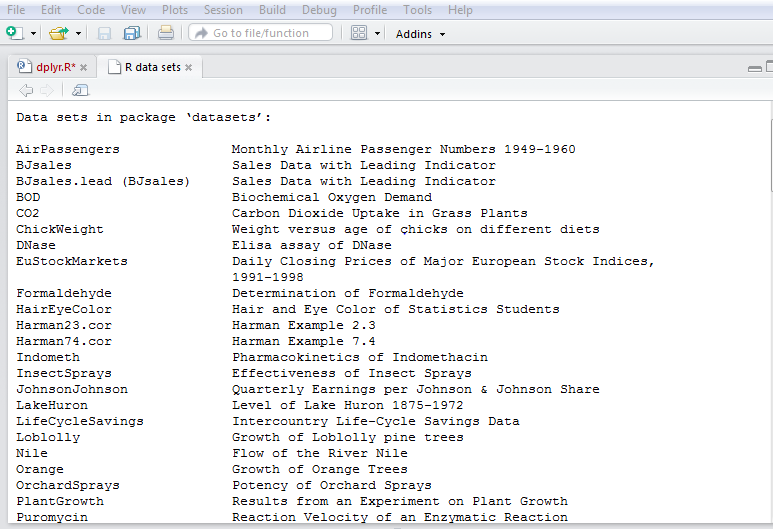We can load “airquality” data by using following command :

data(“airquality”)

We can check the description of “airquality” data by using following code :

?airquality

It will represent the description of dataset , variables and other attributes in Help window.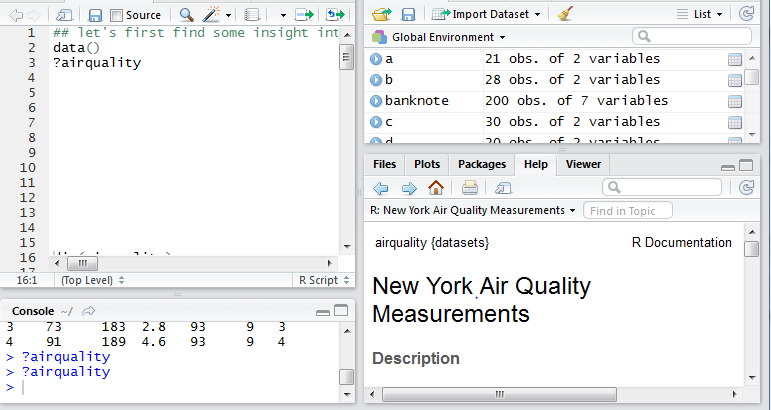We can view “airquality” data by using this code:

View(airquality)

It will open airquality window .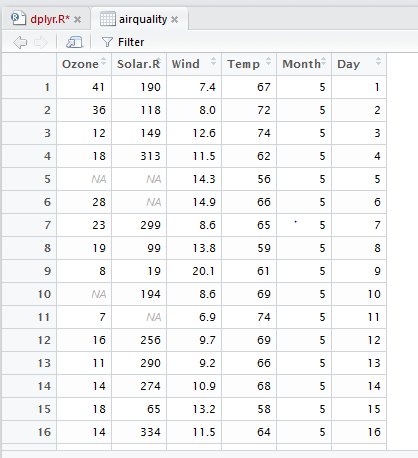Now , we check the attributes of “airquality” data .

We check the dimension of “airquality” data .

dim(airquality)

Output :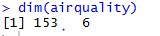It shows 153 rows and 6 columns.

We check the structure of “airquality” data by using following code :

str(airquality)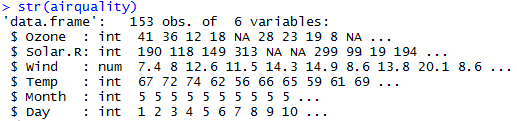It shows various attributes of columns associated with “airquality” data like data type, values etc.

We check the column names of “airquality” data by following code :

names(airquality)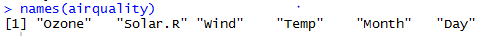We can check out top 6 observations of “airquality” data :

head(airquality)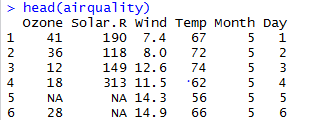Select Ozone , day and Month columns from “airquality” data :

select(airquality, Ozone,Day, Month)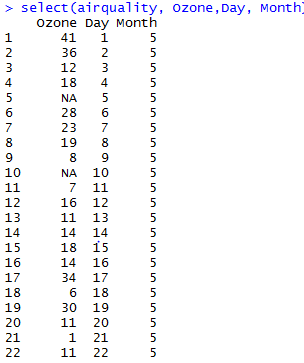Select top three observations of Ozone , Day and Month columns by using following code :

head(object,3)

where “3” represents number of observations to show.

head(select(airquality, Ozone,Day, Month), 3)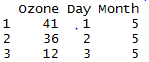It shows Ozone ,Solar.RWind columns of “airquality” data. We used “:” symbol. It will select columns continuously.

head(select(airquality, Ozone:Wind), 3)

or

head(select(airquality, Ozone,Solar.R,Wind), 3)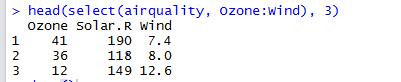We can also unselect columns by using “” sign across column. Here , we do not want to see Solar.R column .

head(select(airquality, -Solar.R), 3)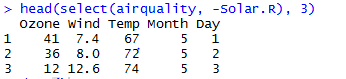We can also unselect multiple columns by:

head(select(airquality, -(Temp:Day)), 3)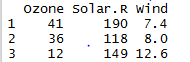Select columns that contains “o” character such as Ozone, Month etc.

head(select(airquality, contains(“o”)), 3)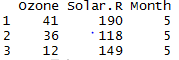filter()

It is used to filter out rows on based of conditions.

Select rows where Month is equal to 9 or Temp(temperature) greater than 90.

filter(airquality, Month == 9, Temp >90)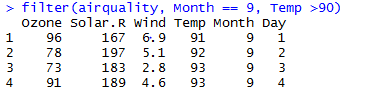We find rows where Day is less than 5 and Solar.R greater than 200 :

filter(airquality, Day <5 & Solar.R >= 200)

Here , we are using “&” symbol to represent “And binary operator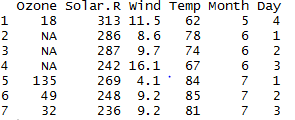We find rows where Day equal to 1 or 2 :

head (filter(airquality, Day %in% c(1,2)),5)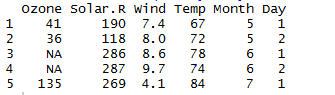We are using “|” symbol to represent “OR” binary operator. We are selecting top 5 observations where Month equals to 8 or Wind less than 5.

head(filter(airquality, Month==8 | Wind < 5), 5)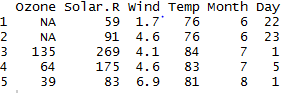We want to select only those rows where Ozone is not missing.

is.na() – Check elements are missing or not

head(filter(airquality, !is.na(Ozone)), 5)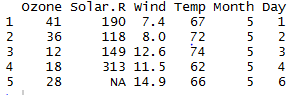arrange()

It is used to sort rows ascending or descending order. When we sort column in our data, it will sort by default in  ascending order.

We sorting rows in the ascending order of Day column . It shows top 6 observations of “airquality” data sorted by Day in ascending order.

head(arrange(airquality, Day))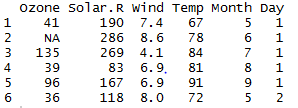We are sorting Temp by descending order . It shows top 6 observations of “airquality” data sorted by Temp in descending order.

head (arrange(airquality, desc(Temp)))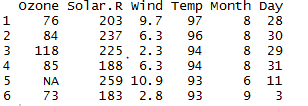We can also sort multiple columns . Here , we sort “airquality” data by Day in  ascending order and then by Month in descending order.

head(arrange(airquality, Day, desc(Month)))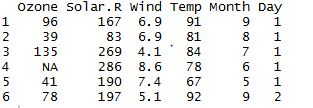mutate()

It adds new variables in dataset.

We add a new variable temp_celsius , which is converting temperature in Celsius from Fahrenheit .

head(mutate(airquality, temp_celsius= (Temp -32)*5/9))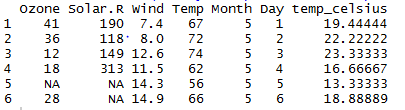We are creating a new dataset “air” to store changes in “airquality” dataset. We create a new variable Dev_Ozone that displays the deviation of Ozone from mean

air<- head (mutate(airquality, Dev_Ozone= Ozone- mean(Ozone, na.rm = TRUE)))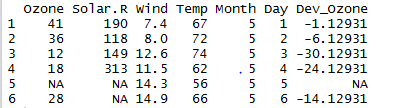We are adding a new variable TempCat which shows values “hot” and “cold” on the basis of Temp value. We have used factor() to change values to factor . If Temp is greater than 80 than it shows “hot” otherwise “cold” . We create a new dataset to store the changes in “airquality” dataset.

air_quality <- mutate(airquality, TempCat = factor((Temp > 80), labels = c(“cold”, “hot”)))

head(air_quality)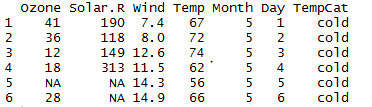group_by()

It allows to split the data set according to categorical variable. We create a new dataset “hot_cold“.

hot_cold <- group_by(air_quality, TempCat)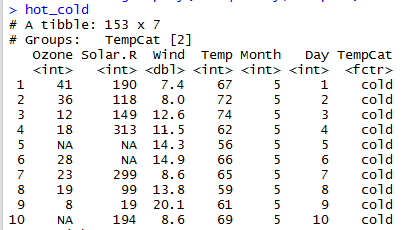summarise()

It is used to summarize data .

We summarise “air_quality” dataset to compute median number of Ozone.

summarise(air_quality, median_Oz = median(Ozone, na.rm = TRUE))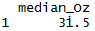Compute minimum and maximum temperature of “air_quality” dataset.

summarise(air_quality, max_temp= max(Temp), min_temp = min(Temp))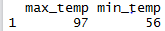We create new dataset “Month_Cat“, which grouped Month column .

Month_Cat <- group_by(air_quality, Month)

Compute average temperature and average temperature in Celsius month-wise.

summarise (Month_Cat, mean_temp = mean(Temp, na.rm = TRUE),

mean_in_celsius = (mean_temp-32)*5/9)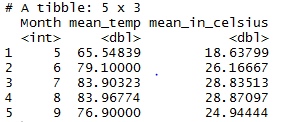summarise_each()

We can apply multiple function to one variable. We have used min and max function . It is used to find minimum and maximum temperature in “airquality” . The syntax of summarise_each function is :

summarise_each( dataset , function , variable)

where function represent mean , min , max etc.

summarise_each(airquality,funs(min_Temp = min, max_Temp = max), Temp)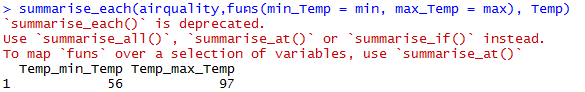We can apply one function to many variables.

summarise_each(airquality,funs(mean),Temp ,Wind )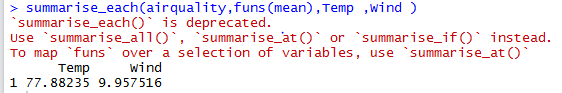We can also summarize result by using summarise_at() . It is used to summarize data by applying many functions on many variables.

summarise_at(airquality,vars(Temp , Solar.R),funs(n(),mean(.,na.rm = T)))

Here ,  vars() – represent variables list

n() – count number of observation of variables

mean(.,na.rm=T) – find average of variable .

where “.” represent variable Temp and Solar.R

Output :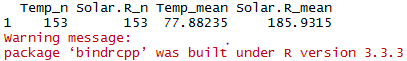We can also apply our own function(custom)  to summarize data.

I have create a function :-

function(x) var(x-mean(x) )

Where var represents variance and mean represent average .

var(x-mean(x)) shows variance of difference between x and average value of x .

We compute variance of difference between Temp and average value of Temp :

summarise_at(airquality,vars(Temp), function(x) var(x – mean(x)))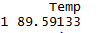rename()

It is used to rename the variables in dataset.

We can rename variable “Temp” to “Temperature“.

air_quality1<-rename(airquality,Temperature=Temp)

head(air_quality1)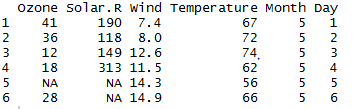count()

The count function counts observation based on a group . We are counting number of observations in each month .

count(airquality,Month)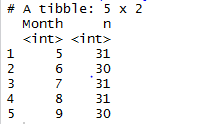Pipe Operator %>%

dplyr” package imports pipe operator from another package called “magrittr” . It is used to pipe the output from one function to the input of another function . Pipe is used to connect one function output to input of other function .

airquality %>%

select(Ozone, Wind) %>%

head

Here , we first pass “airqaulity” data to select function to select Ozone and Wind column. Then , we pass output to head function to show only top 6 observations.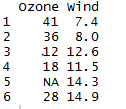We want to find the average number of Ozone in last 6 days of May Month.

First , we pass the “airquality” dataset to filter out Month equals to May and Day greater than 25. We pass the output of filter function to summarise function to find average of Ozone .

air_quality %>%

filter(Month== 5 & Day > 25) %>%

summarise(Ozone = mean(Ozone, na.rm=TRUE))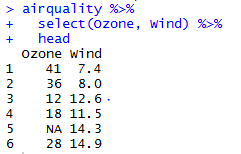Compute average temperature of May to August months .

We are grouping Month column and then we filter out Month where it lies between 4 and 8 . We find average temperature of by months.

airquality %>%

group_by(Month) %>%

filter(Month > 4 & Month <=8) %>%

summarise(mean=mean(Temp, na.rm=TRUE))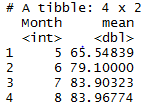We re-arrange the data set and select all rows that display only hot days in the Temperature Category(TEmpCat) .

We create a new dataset air_quality which adds a new variable TempCat . TempCat shows value as hot or cold . If Temp is greater than 80 then it shows hot otherwise cold.

We re-arrange air_quality by sorting Temp in descending order and then Day in ascending order. We pass the output to filter function to select only hot in TempCat . We pass the output to head function to show top 6 observations.

air_quality <- mutate(airquality, TempCat = factor((Temp > 80), labels = c(“cold”, “hot”)))

air_quality %>%

arrange(desc(Temp), Day) %>%

filter(TempCat %in% (“hot”)) %>%

head

We pass air_quality data to group_by function to grouping TemCat variable. Then we pass the output to summarise function to find average of Solar.R and maximum value of Wind on TempCat values. It shows mean and maximum value of Solar.R and Wind respectively of hot and cold temperature.

air_quality %>%

group_by(TempCat)%>%

summarise(Solar.R= mean(Solar.R, na.rm=TRUE),

Wind=max(Wind, na.rm=TRUE))

For each Month , calculate minimum , maximum and average value of column names contains “o” value. The column names Solar.R and Ozone columns contains “o” values. For each month, we find out minimum , maximum and average value of Ozone and Solar.R.

airquality%>%

group_by(Month)%>%

summarise_each(funs(mean(.,na.rm=T),min(.,na.rm=T),max(.,na.rm=T)),matches(“o”))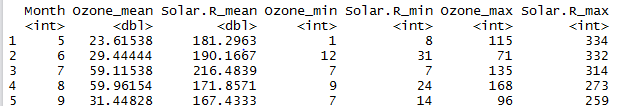Previous Article